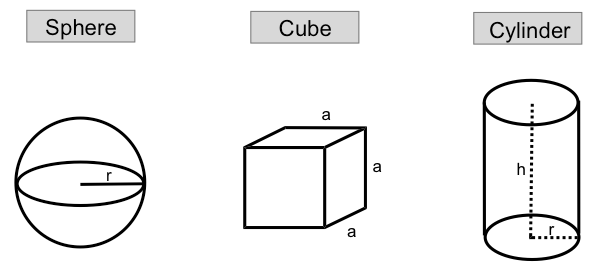Clutch Prep is now a part of Pearson
Ch.1 - Intro to General ChemistryWorksheetSee all chapters

# Density of Geometric Objects

See all sections
Sections
Classification of Matter
Physical & Chemical Changes
Chemical Properties
Physical Properties
Intensive vs. Extensive Properties
Temperature
Scientific Notation
SI Units
Metric Prefixes
Significant Figures
Significant Figures: Precision in Measurements
Significant Figures: In Calculations
Conversion Factors
Dimensional Analysis
Density
Density of Geometric Objects
Density of Non-Geometric Objects

The density of geometric objects generally includes spheres, cubes and cylinders.

###### Calculating Density

Concept #1: When given the mass of a geometric object you can relate it to its volume and density.Example #1: The density of silver is 10.5 g/cm3. What is the mass (in kilograms) of a cube of silver that measures 0.56 m on each side?

Practice: A copper wire (density = 8.96 g/cm3) has a diameter of 0.32 mm. If a sample of this copper wire has a mass of 21.7 g, how long is the wire?

Practice: If the density of a certain spherical atomic nucleus is 1.0 x 1014 g/cm3 and its mass is 3.5 x 10-23 g, what is the radius in angstroms? (Å= 10−10 m)